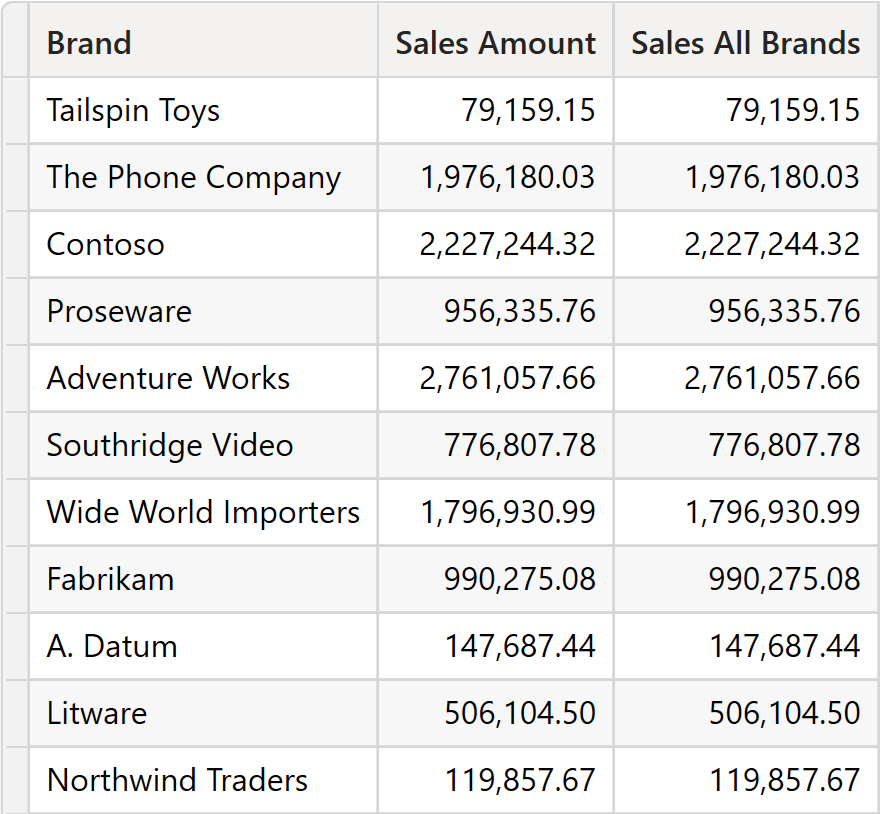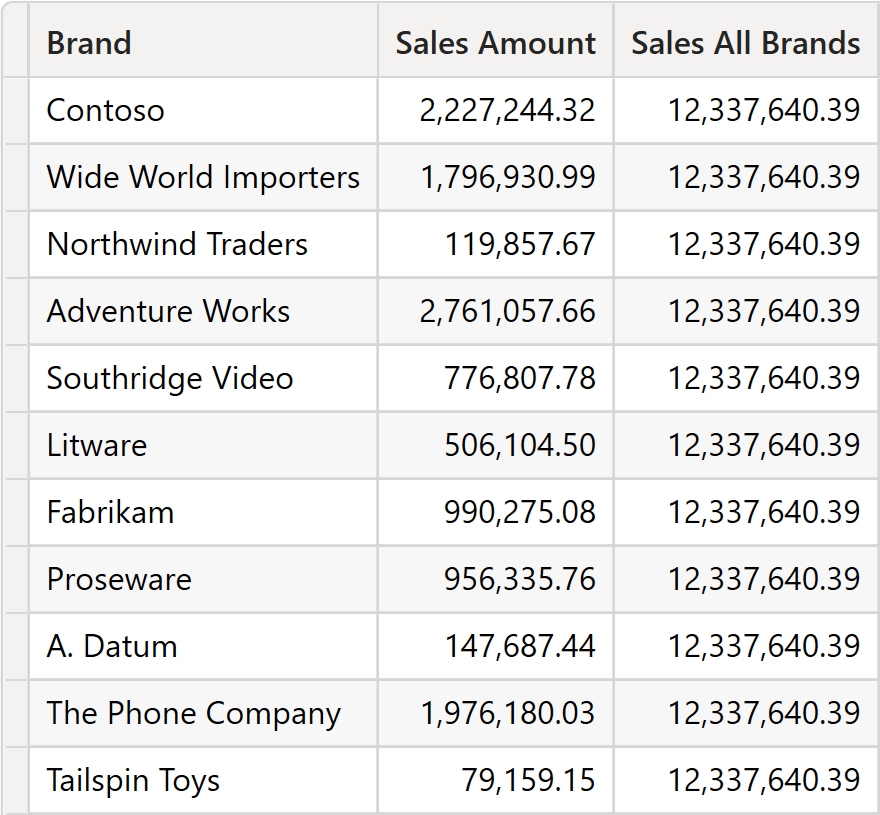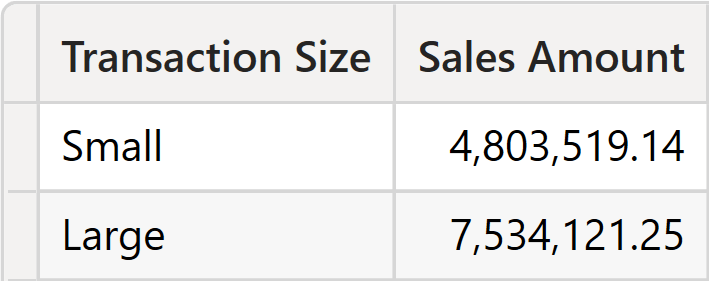DAX offers a rich set of functions, some of which overlap in their functionalities. Among the many, two functions perform grouping: SUMMARIZE and GROUPBY. These are not the only two: SUMMARIZECOLUMNS and GROUPCROSSAPPLY perform similar operations. However, the article is about SUMMARIZE and GROUPBY, as the other functions have many more functionalities, so a comparison would be unfair.

To make a long story short: GROUPBY should be used to group by local columns, columns created on the fly by DAX functions. SUMMARIZE should be used to group by model and query columns. Be mindful that both functions support both scenarios: both functions can group by model and local columns. However, using the wrong function translates into a strong decrease in performance.

Let us now elaborate on how these functions work to provide more technical information about the previous statement.

## Introducing SUMMARIZE

SUMMARIZE performs two operations: grouping by and adding new local columns. We already wrote about SUMMARIZE in a long and deeply technical article: All the secrets of SUMMARIZE. In that article, we describe the behavior of SUMMARIZE and why it should never be used to compute new local columns. Specifically, SUMMARIZE implements clustering, a grouping technique that – despite being very powerful – may lead to unexpected results and poor performance.

However, for the sake of this comparison, we will use SUMMARIZE to compute new columns to describe its peculiar behavior.

When used with simple examples, SUMMARIZE behaves nicely, pushing the grouping operation down to the storage engine. For example, the following code works just fine, producing the expected storage engine queries:

```EVALUATE
SUMMARIZE (
Sales,
'Product'[Brand],
"Sales Amount", [Sales Amount]
)
```

SUMMARIZE scans Sales, groups it by Product[Brand], and produces the sales amount by brand. The storage engine query is the following:

```WITH
\$Expr0 := ( PFCAST ( 'Sales'[Quantity] AS INT ) * PFCAST ( 'Sales'[Net Price] AS INT ) )
SELECT
'Product'[Brand],
SUM ( @\$Expr0 )
FROM 'Sales'
LEFT OUTER JOIN 'Product'
ON 'Sales'[ProductKey]='Product'[ProductKey];
```

However, this simple behavior is easily lost as soon as the code of the measure being executed becomes a bit more complex. Indeed, as we mentioned SUMMARIZE performs its calculation using a special technique named clustering, described in the article cited earlier. Look at the following code:

```EVALUATE
SUMMARIZE (
Sales,
'Product'[Brand],
"Sales Amount", [Sales Amount],
"Sales All Brands",
CALCULATE (
[Sales Amount],
REMOVEFILTERS ( Product[Brand] )
)
)
```

It would be reasonable to expect Sales All Brands to produce the grand total of sales, as CALCULATE removes the only filter present in the filter context. However, this speculation does not consider clustering. Because of clustering, the filter placed by SUMMARIZE affects all columns of the expanded Sales table, resulting in this strange outcome.As you see, Sales All Brands repeats the same values as Sales Amount. A different data distribution or the presence of duplicated rows might result in different values. Moreover, because of clustering, as soon as the measure to aggregate is non-trivial, SUMMARIZE needs to materialize the full table. To compute Sales All Brands, this is one of the VertiPaq queries being executed:

```WITH
\$Expr0 := ( PFCAST ( 'Sales'[Quantity] AS INT ) * PFCAST ( 'Sales'[Net Price] AS INT ) )
SELECT
'Sales'[Order Number],
'Sales'[Line Number],
'Sales'[Order Date],
'Sales'[Delivery Date],
'Sales'[CustomerKey],
'Sales'[StoreKey],
'Sales'[ProductKey],
'Sales'[Quantity],
'Sales'[Unit Price],
'Sales'[Net Price],
'Sales'[Unit Cost],
'Sales'[Currency Code],
'Sales'[Exchange Rate],
SUM ( @\$Expr0 )
FROM 'Sales';
```

Please note that RowNumber is not part of the query, so the granularity of the datacache is not exactly the granularity of Sales, as is the case with GROUPBY. However, because all the columns of the table are used as group-by columns, the size is often very relevant.

The same query, using SUMMARIZE and ADDCOLUMNS, produces the expected result:

```EVALUATE
SUMMARIZE (
Sales,
'Product'[Brand]
),
"Sales Amount", [Sales Amount],
"Sales All Brands",
CALCULATE (
[Sales Amount],
ALL ( Product[Brand] )
)
)
```

Here is the result.Thanks to clustering, SUMMARIZE can perform grouping by local columns too. The following query works just fine, despite grouping by a local column:

```EVALUATE
SUMMARIZE (
Sales,
"Transaction Size",
IF (
Sales[Quantity] > 3,
"Large",
"Small"
)
),
[Transaction Size],
"Sales Amount", [Sales Amount]
)
```

The result shows the sales amount grouped by transaction size.However, remember that despite the query working from a syntactical and semantical point of view, its result is computed by using clustering. Clustering produces surprising results in multiple scenarios, and it is a functionality that creates more problems than solutions. Besides, also in this case, the calculation would require the materialization of the entire Sales table.

## Introducing GROUPBY

GROUPBY groups a table by one of its columns. The column can be a model column or a local column. However, its behavior is very different from that of SUMMARIZE. GROUPBY does not even try to push the calculation down to the storage engine: the entire calculation happens in the formula engine after materializing the table. GROUPBY can also add new columns to its result. However, because of its behavior, new columns need to be computed as simple aggregations of the columns in the table being grouped using the CURRENTGROUP special function.

As an example, let us look at the following code:

```EVALUATE
GROUPBY (
Sales,
'Product'[Brand],
"Sales Amount",
SUMX (
CURRENTGROUP (),
Sales[Quantity] * Sales[Net Price]
)
)
```

GROUPBY scans the Sales table and groups it by Product[Brand]. To perform the grouping, DAX materializes the required columns of Sales in a datacache, which is then processed by the formula engine. Indeed, the query executes this code:

```SELECT
'Product'[Brand],
'Sales'[RowNumber],
'Sales'[Quantity],
'Sales'[Net Price]
FROM 'Sales'
LEFT OUTER JOIN 'Product'
ON 'Sales'[ProductKey]='Product'[ProductKey];
```

Out of Sales, DAX retrieves Sales[Quantity], Sales[Net Price], and Product[Brand]. The presence of Sales[RowNumber] guarantees that all the rows are retrieved – otherwise, VertiPaq itself would perform a grouping operation.

The result is a table with the same number of rows as Sales, thus potentially very large. The table is scanned by the formula engine, which splits it into clusters based on Product[Brand], and then for each cluster it computes the sum of the multiplication of Sales[Quantity] by Sales[Net Price].

A strong limitation of GROUPBY is that the expression used while iterating CURRENTGROUP cannot involve a context transition. This limitation makes using existing measures as part of the iteration impossible. As you might have noticed, we had to rewrite the code of Sales Amount in the example.

Despite seeming slow, GROUPBY is the only DAX function that can perform grouping and calculations over a table with no lineage. For example, the following query groups a local table by one of its columns, and GROUPBY is the only function capable of performing the operation:

```EVALUATE
VAR TableToGroup =
SELECTCOLUMNS (
{
( "A", 1 ),
( "A", 2 ),
( "B", 3 ),
( "B", 4 )
},
"Group", [Value1],
"Value", [Value2]
)
RETURN
GROUPBY (
TableToGroup,
[Group],
"Result",
SUMX (
CURRENTGROUP (),
[Value]
)
)
```

GROUPBY is the right function to use when you produce a small table using other DAX functions, and then you need to perform grouping by one of the columns performing a simple aggregation on a row-by-row basis.

## Choosing the right function

As you have seen, SUMMARIZE works well when you need to group by columns in the model. Despite having the capability of grouping also by local columns, it uses clustering, and its results are mostly unexpected. GROUPBY does not use clustering. However, it poses a very strong limitation: it always materializes the table it needs to group. As such, it is not the best option to perform grouping by model columns, whereas the pair ADDCOLUMNS/SUMMARIZE mostly produces more efficient code.

However, when there is the need to group a small temporary table by a local column, GROUPBY is the best function because it does the job without relying on clustering.

A wise DAX developer chooses the right function for the job, often mixing SUMMARIZE, ADDCOLUMNS, and GROUPBY to obtain the best performance and the correct results. Let us elaborate on this with an example. Previously, we showed you this code:

```EVALUATE
SUMMARIZE (
Sales,
"Transaction Size",
IF (
Sales[Quantity] > 3,
"Large",
"Small"
)
),
[Transaction Size],
"Sales Amount", [Sales Amount]
)
```

This query uses SUMMARIZE, therefore clustering. It executes two VertiPaq queries. The first one basically materializes Sales:

```SELECT
'Sales'[Order Number],
'Sales'[Line Number],
'Sales'[Order Date],
'Sales'[Delivery Date],
'Sales'[CustomerKey],
'Sales'[StoreKey],
'Sales'[ProductKey],
'Sales'[Quantity],
'Sales'[Unit Price],
'Sales'[Net Price],
'Sales'[Unit Cost],
'Sales'[Currency Code],
'Sales'[Exchange Rate]
FROM 'Sales';
```

The second storage engine query uses the results of the first one to build a massive filter over Sales:

```WITH
\$Expr0 := ( PFCAST ( 'Sales'[Quantity] AS INT ) * PFCAST ( 'Sales'[Net Price] AS INT ) )
SELECT
'Sales'[Order Number],
'Sales'[Line Number],
'Sales'[Order Date],
'Sales'[Delivery Date],
'Sales'[CustomerKey],
'Sales'[StoreKey],
'Sales'[ProductKey],
'Sales'[Quantity],
'Sales'[Unit Price],
'Sales'[Net Price],
'Sales'[Unit Cost],
'Sales'[Currency Code],
'Sales'[Exchange Rate],
SUM ( @\$Expr0 )
FROM 'Sales'
WHERE
( 'Sales'[Exchange Rate], 'Sales'[Currency Code], 'Sales'[Unit Cost], 'Sales'[Net Price], 'Sales'[Unit Price], 'Sales'[Quantity],
'Sales'[ProductKey], 'Sales'[StoreKey], 'Sales'[CustomerKey], 'Sales'[Delivery Date], 'Sales'[Order Date],
'Sales'[Line Number], 'Sales'[Order Number] )
IN { ( 1.000000, 'USD', 1227800, 2536500, 2670000, 1, 1507, 999999, 1573592, 43818.000000, 43816.000000, 1, 363800 ) ,
( 0.914500, 'EUR', 1677300, 2928100, 3290000, 2, 241, 999999, 587554, 43739.000000, 43736.000000, 2, 355804 ) ,
( 0.902900, 'EUR', 676000, 1470000, 1470000, 1, 668, 340, 884269, 43693.000000, 43693.000000, 1, 351503 ) ,
( 1.335200, 'CAD', 322500, 701300, 701300, 3, 1707, 999999, 278457, 43473.000000, 43472.000000, 1, 329404 ) ,
( 1.000000, 'USD', 1480780, 3220000, 3220000, 3, 1410, 999999, 1582937, 43095.000000, 43090.000000, 0, 291214 ) ,
( 1.297600, 'CAD', 3214400, 6990000, 6990000, 1, 405, 80, 326829, 43836.000000, 43836.000000, 2, 365800 ) ,
( 1.000000, 'USD', 300800, 513300, 590000, 2, 501, 999999, 1540547, 43818.000000, 43813.000000, 1, 363503 ) ,
( 1.000000, 'USD', 186500, 364950, 405500, 6, 79, 450, 1665181, 43239.000000, 43239.000000, 0, 306110 ) ,
( 1.310000, 'CAD', 1520800, 4590000, 4590000, 4, 569, 100, 384389, 43407.000000, 43407.000000, 0, 322905 ) ,
( 0.875900, 'EUR', 1379600, 3000000, 3000000, 1, 1449, 999999, 590077, 43410.000000, 43406.000000, 0, 322800 )
..[13,915 total tuples, not all displayed]};
```

Despite working very fast on our sample model, these two queries may be extremely heavy and slow in a real-world example with tens of millions of rows in Sales.

The same query, expressed using GROUPBY, is likely to be more efficient:

```EVALUATE
GROUPBY (
Sales,
"Transaction Size",
IF (
Sales[Quantity] > 3,
"Large",
"Small"
)
),
[Transaction Size],
"Sales Amount",
SUMX (
CURRENTGROUP (),
Sales[Quantity] * Sales[Net Price]
)
)
```

The materialization level is smaller, even though we cannot use the base measure Sales Amount. The only VertiPaq query being executed is the following:

```SELECT
'Sales'[RowNumber],
'Sales'[Quantity],
'Sales'[Net Price]
FROM 'Sales';
```

However, the granularity of this datacache is the same as Sales, and it would be a serious issue on large models.

Obtaining better performance requires combining the two functions and changing our perspective. We first group by Sales[Quantity], generating a very small table using ADDCOLUMNS and SUMMARIZE. The table contains only 10 rows. We then add the Transaction Size column, and we finally use GROUPBY to group the table with 10 rows in the two Transaction Size clusters:

```EVALUATE
GROUPBY (
SUMMARIZE (
Sales,
Sales[Quantity]
),
"@Sales", [Sales Amount],
"Transaction Size",
IF (
Sales[Quantity] > 3,
"Large",
"Small"
)
),
[Transaction Size],
"Sales Amount",
SUMX (
CURRENTGROUP (),
[@Sales]
)
)
```

This DAX query executes only two storage engine queries. The first query groups sales amount by quantity:

```WITH
\$Expr0 := ( PFCAST ( 'Sales'[Quantity] AS INT ) * PFCAST ( 'Sales'[Net Price] AS INT ) )
SELECT
'Sales'[Quantity],
SUM ( @\$Expr0 )
FROM 'Sales';
```

The second VertiPaq query just retrieves the different values of Sales[Quantity]:

```SELECT
'Sales'[Quantity]
FROM 'Sales';
```

Most of the calculation has been pushed down to the storage engine; the materialization level is negligible, and this last DAX query will be extremely fast, even on large databases.

## Conclusions

Knowing the details of functions, how they are implemented, and their expected usage is an important skill for anybody serious about DAX. In this article, we covered the difference between GROUPBY and SUMMARIZE. However, DAX has many more hidden details worth learning about.

Using the wrong function may either produce unexpected results or inefficient queries. The more you learn about DAX, the better your code will become.

SUMMARIZECreates a summary of the input table grouped by the specified columns.

`SUMMARIZE ( <Table> [, <GroupBy_ColumnName> [, [<Name>] [, [<Expression>] [, <GroupBy_ColumnName> [, [<Name>] [, [<Expression>] [, … ] ] ] ] ] ] ] )`

GROUPBYCreates a summary the input table grouped by the specified columns.

`GROUPBY ( <Table> [, <GroupBy_ColumnName> [, [<Name>] [, [<Expression>] [, <GroupBy_ColumnName> [, [<Name>] [, [<Expression>] [, … ] ] ] ] ] ] ] )`

SUMMARIZECOLUMNSCreate a summary table for the requested totals over set of groups.

`SUMMARIZECOLUMNS ( [<GroupBy_ColumnName> [, [<FilterTable>] [, [<Name>] [, [<Expression>] [, <GroupBy_ColumnName> [, [<FilterTable>] [, [<Name>] [, [<Expression>] [, … ] ] ] ] ] ] ] ] ] )`

CALCULATEContext transition

Evaluates an expression in a context modified by filters.

`CALCULATE ( <Expression> [, <Filter> [, <Filter> [, … ] ] ] )`

ADDCOLUMNSReturns a table with new columns specified by the DAX expressions.

`ADDCOLUMNS ( <Table>, <Name>, <Expression> [, <Name>, <Expression> [, … ] ] )`

CURRENTGROUPAccess to the (sub)table representing current group in GroupBy function. Can be used only inside GroupBy function.

`CURRENTGROUP ( )`